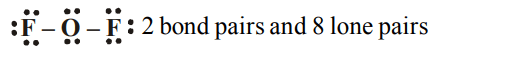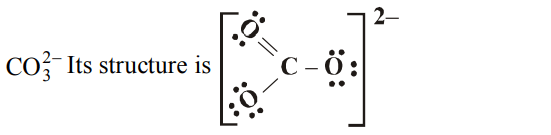## Chemical Bonding and Molecular Structure Questions and Answers Part-6

1. According to VSEPR theory, the most probable shape of the molecule having 4 electron pairs in the outershell of the central atom is
a) linear
b) tetrahedral
c) hexahedral
d) octahedral

Explanation: Tetrahedral

2. The geometry of $CIO_3^-$ ion according to Valence Shell Electron Pair Repulsion (VSEPR) theory will be
a) planar triangular
b) pyramidal
c) tetrahedral
d) square planar

Explanation: Hybridisation is sp3 and shape pyramidal

3. Among the following ions, the $p\pi-d\pi$   overlap could be present in
a) $NO_3^-$
b) $PO_4^{3-}$
c) $CO_3^{2-}$
d) $NO_2^-$

Explanation: Hybridisation in $PO_4^{3-}$ = ½ [5 + 0 + 3 –0] = 4 sp3 . In $\pi$ bonding only d orbital of P, p orbital of O can be involved. Since hybrid atomic orbitals do not form $\pi$ bond.

4. In $NO_3^-$ ion, number of bond pairs and lone pairs of electrons on nitrogen atom are
a) 2, 2
b) 3,1
c) 1,3
d) 4,0

Explanation:5. In $OF_{2}$, number of bond pairs and lone pairs of electrons are respectively
a) 2,6
b) 2,8
c) 2,10
d) 2,9

Explanation:6. The compound containing co-ordinate bond is
a) $H_{2}SO_{4}$
b) $O_{3}$
c) $SO_{3}$
d) All of these

Explanation:7. Sulphuric acid provides a simple example of
a) co-ordinate bonds
b) non-covalent compound
c) covalent ion
d) non - covalent ion

Explanation: Coordinate bond

8. Which of the following does not contain coordinate bond ?
a) $BH_4^-$
b) $NH_4^+$
c) $CO_3^{2-}$
d) $H_{3}O^{+}$

Explanation:9. The maximum number of water molecules that one water molecule can hold through hydrogen bonding is
a) 2
b) 4
c) 6
d) 8

10. $NH_{3}$ has abnormally high boiling point because it has
c) $sp^{3}$ - Hybridization